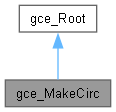Open CASCADE Technology  7.7.0.dev

# gce_MakeCirc Class Reference

This class implements the following algorithms used to create Circ from gp. More...

`#include <gce_MakeCirc.hxx>`

Inheritance diagram for gce_MakeCirc:[legend]

## Public Member Functions

gce_MakeCirc (const gp_Ax2 &A2, const Standard_Real Radius)
A2 locates the circle and gives its orientation in 3D space. Warnings : It is not forbidden to create a circle with Radius = 0.0 The status is "NegativeRadius" if Radius < 0.0. More...

gce_MakeCirc (const gp_Circ &Circ, const Standard_Real Dist)
Makes a Circ from gp <TheCirc> coaxial to another Circ <Circ> at a distance <Dist>. If Dist is greater than zero the result is encloses the circle <Circ>, else the result is enclosed by the circle <Circ>. More...

gce_MakeCirc (const gp_Circ &Circ, const gp_Pnt &Point)
Makes a Circ from gp <TheCirc> coaxial to another Circ <Circ> and passing through a Pnt2d <Point>. More...

gce_MakeCirc (const gp_Pnt &P1, const gp_Pnt &P2, const gp_Pnt &P3)
Makes a Circ from gp <TheCirc> passing through 3 Pnt2d <P1>,<P2>,<P3>. More...

gce_MakeCirc (const gp_Pnt &Center, const gp_Dir &Norm, const Standard_Real Radius)
Makes a Circ from gp <TheCirc> with its center. More...

gce_MakeCirc (const gp_Pnt &Center, const gp_Pln &Plane, const Standard_Real Radius)
Makes a Circ from gp <TheCirc> with its center. More...

gce_MakeCirc (const gp_Pnt &Center, const gp_Pnt &Ptaxis, const Standard_Real Radius)
Makes a Circ from gp <TheCirc> with its center. More...

gce_MakeCirc (const gp_Ax1 &Axis, const Standard_Real Radius)
Makes a Circ from gp <TheCirc> with its center. More...

const gp_CircValue () const
Returns the constructed circle. Exceptions StdFail_NotDone if no circle is constructed. More...

const gp_CircOperator () const

operator gp_Circ () constPublic Member Functions inherited from gce_Root
Standard_Boolean IsDone () const
Returns true if the construction is successful. More...

gce_ErrorType Status () const
Returns the status of the construction: More...

## Additional Inherited MembersProtected Attributes inherited from gce_Root
gce_ErrorType TheError

## Detailed Description

This class implements the following algorithms used to create Circ from gp.

• Create a Circ coaxial to another and passing though a point.
• Create a Circ coaxial to another at the distance Dist.
• Create a Circ passing through 3 points.
• Create a Circ with its center and the normal of its plane and its radius.
• Create a Circ with its center and its plane and its radius.
• Create a Circ with its axis and radius.
• Create a Circ with two points giving its axis and its radius.
• Create a Circ with is Ax2 and its Radius.

## ◆ gce_MakeCirc() [1/8]

 gce_MakeCirc::gce_MakeCirc ( const gp_Ax2 & A2, const Standard_Real Radius )

A2 locates the circle and gives its orientation in 3D space. Warnings : It is not forbidden to create a circle with Radius = 0.0 The status is "NegativeRadius" if Radius < 0.0.

## ◆ gce_MakeCirc() [2/8]

 gce_MakeCirc::gce_MakeCirc ( const gp_Circ & Circ, const Standard_Real Dist )

Makes a Circ from gp <TheCirc> coaxial to another Circ <Circ> at a distance <Dist>. If Dist is greater than zero the result is encloses the circle <Circ>, else the result is enclosed by the circle <Circ>.

## ◆ gce_MakeCirc() [3/8]

 gce_MakeCirc::gce_MakeCirc ( const gp_Circ & Circ, const gp_Pnt & Point )

Makes a Circ from gp <TheCirc> coaxial to another Circ <Circ> and passing through a Pnt2d <Point>.

## ◆ gce_MakeCirc() [4/8]

 gce_MakeCirc::gce_MakeCirc ( const gp_Pnt & P1, const gp_Pnt & P2, const gp_Pnt & P3 )

Makes a Circ from gp <TheCirc> passing through 3 Pnt2d <P1>,<P2>,<P3>.

## ◆ gce_MakeCirc() [5/8]

 gce_MakeCirc::gce_MakeCirc ( const gp_Pnt & Center, const gp_Dir & Norm, const Standard_Real Radius )

Makes a Circ from gp <TheCirc> with its center.

and the normal of its plane <Norm> and its radius <Radius>.

## ◆ gce_MakeCirc() [6/8]

 gce_MakeCirc::gce_MakeCirc ( const gp_Pnt & Center, const gp_Pln & Plane, const Standard_Real Radius )

Makes a Circ from gp <TheCirc> with its center.

and the normal of its plane <Plane> and its radius <Radius>.

## ◆ gce_MakeCirc() [7/8]

 gce_MakeCirc::gce_MakeCirc ( const gp_Pnt & Center, const gp_Pnt & Ptaxis, const Standard_Real Radius )

Makes a Circ from gp <TheCirc> with its center.

and a point <Ptaxis> giving the normal of its plane <Plane> and its radius <Radius>.

## ◆ gce_MakeCirc() [8/8]

 gce_MakeCirc::gce_MakeCirc ( const gp_Ax1 & Axis, const Standard_Real Radius )

Makes a Circ from gp <TheCirc> with its center.

and its radius <Radius>. Warning The MakeCirc class does not prevent the construction of a circle with a null radius. If an error occurs (that is, when IsDone returns false), the Status function returns:
• gce_Negative Radius if:
• Radius is less than 0.0, or
• Dist is less than 0.0 and the absolute value of Dist is greater than the radius of Circ;
• gce_IntersectionError if the points P1, P2 and P3 are collinear, and the three are not coincident;
• gce_ConfusedPoints if two of the three points P1, P2 and P3 are coincident; or
• gce_NullAxis if Center and Ptaxis are coincident.

## ◆ Operator()

 const gp_Circ& gce_MakeCirc::Operator ( ) const

## ◆ operator gp_Circ()

 gce_MakeCirc::operator gp_Circ ( ) const

## ◆ Value()

 const gp_Circ& gce_MakeCirc::Value ( ) const

Returns the constructed circle. Exceptions StdFail_NotDone if no circle is constructed.

The documentation for this class was generated from the following file: